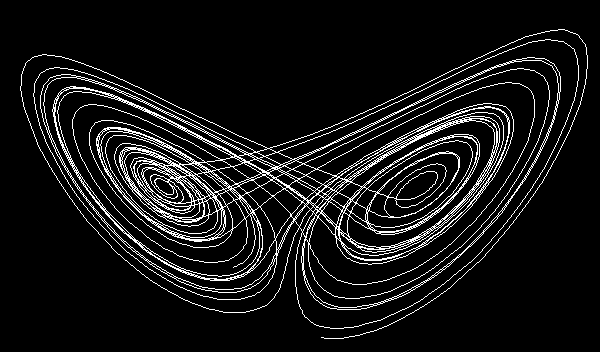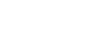The Sound of Mathematics - Recursion

RECURSION

Lorenz's Butterfly

Lorenz's butterfly is a so called strange attractor defined by the differential equations

dx/dt = 10(y - x)
dy/dt = x(28 - z) - y
dz/dt = xy - 8z/3.

I used iterative equations to calculate the values of the three above equations where the change of t = 0.01 and the initial values x0 = 2, y0 = 3 and z0 = 5. On a xz-plane, the 3500 iterations used for the piece of music looks like this:The piece starts at the tail you see at the bottom of the picture and every fifth value of the three respective equations were mapped simultaneously to three parts. The procedure was the same for all three of them: The values were rounded off to the nearest integer. For values in the interval -7 to 8, excluding the zero (which is a pause), 1 maps to the E of the E phrygian mode (of three consecutive octaves for the respective parts), and the other values are ordered accordingly. For all other values, if the number is odd - descend a scale step, if the value is even - ascend a scale step. The number of equal consecutive values maps to that number of multiples of a unit note value. 699 values of each of the equations were used.

Playing time: 2' 27".

The Fibonacci Sequence

The Fibonacci sequence, 1 1 2 3 5 8 13 21 34... etc. is generated by the recursive formula fn = fn-1 + fn-2, for n = 2, 3, 4, ... and f0 =1, f1 = 1. So each Fibonacci number is the sum of the previous two Fibonacci numbers (apart from the two initial values).

The piece of music uses the first eight Fibonacci numbers. For each new number, the note value is alternatingly decreased by a factor 1/2 or 1/3. The pitch is ascending one step up an E minor chord. After four steps, the pitch goes back to the tonic E again, but one octave up, and so on... Each new part is played over the two previous parts.

Playing time: 3' 40".

The Golden String

The golden string is an infinite non-repetitive binary string. It's a fractal in the sense that it is self similar everywhere. One way to generate it is as follows: Call the initial steps s0 = 0 and s1 = 1. For n = 2, 3, 4, ... the step sn is the concatenation of sn-1 and sn-2. So the first steps of the forming of the golden string are 0, 1, 10, 101, 10110, 10110101, 1011010110110, 101101011011010110101... and as n goes towards infinity, sn is the golden string.

Basically, there are two parts in the piece of music:

1) Digits of the golden string taken five at a time (moving one digit to the right for the next five digits). The possible five down to two digit permutations were mapped to a tone of the E whole tone scale as shown in the table. The strings of five digits maps to the first tone of the five tone phrases you'll hear in the piece. The first four digits maps to the second tone, and so on... The first 162 digits of the golden string were used.

 Pitch E1 F# G# A# C D E2 F# G# A# String 01011 01101 10101 10110 11010 11011 0101 0110 1010 1011 1101 010 011 101 110 01 10 11 0 1

2) The ratio between the number of 1's and the number of 0's in the golden string is

(Ö5 + 1) / 2 = 1.61803398874989484820458683436563811772030917980576...

This value is known as the golden mean. The digit 0 was mapped to a pause, digits 1 - 9 were mapped to the tones E1 F F# G G# A# C D E2 respectively. The tones are taken one octave higher when the first part has reached its 101st digit. Equal consecutive tones were tied. The first 633 digits of the golden mean was used.

Playing time: 2' 06".

The Sierpinski Triangle

The Sierpinski triangle is a fractal and the most striking thing about it is that it has no area. To form it, start with a solid triangle. At every step s (first step is 0), reduce the area by erasing 3s upside down triangles with the area (1/4)s+1 of the initial triangle. After infinitely many steps the area is equal to 0.In the piece below, the initial solid triangle was constructed by 16 notes (6 pitches of the E lydian scale) within 4 octaves over 32 bars by adding a whole note at every bar while ascending to the tip of it and then subtracting a whole note at every bar while descending. At every step, notes representing upside down triangles are erased from this solid triangle. Due to the "resolution" I used, it was only possible to go on to the third step. So the music produces the third step of the forming of the Sierpinski triangle.

Playing time: 2' 07".

INDEX